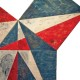# The Groove of Math

## or in other words, the Devil is in the Details# Filling the Details #2: Representing 2-homology classes of a 4-manifold.

For the second article under the FtD tag, I’ve chosen a nice proposition which bothered me some times ago. It’s intuitively easy to believe but I found a scarce amount of details spelled out in the proofs one can find on the classical references. So here we have the following result:

Proposition: Every$2$-homology class of a smooth 4-manifold is generated by a surface

The classical proof goes as follows

Proof: (from Kirby’s Topology of$4$-Manifolds Theorem 1.1 page 20) There is an isomorphism:$H^2(M, \mathbb{Z})\cong [M,\mathbb{C}P^{\infty}]$

so letting$\hat{\alpha}$ being the Poincaré dual of a chosen$\alpha \in H_2(M, \mathbb{Z})$, there is an homotopy class of maps$[f]\colon M \to \mathbb{C}P^{\infty}$ corresponding to$\hat{\alpha}$. By cellular approximation, we can homotopy (a representative of)$f$ in order to obtain a map$f\colon M \to \mathbb{C}P^2$. In fact the 4-skeleton of$\mathbb{C}P^{\infty}$ is$\mathbb{C}P^{2}$ and cellular approximation tells you that the image of$M$ lies there. Make$f$ smoothly transverse to$\mathbb{C}P^1\subset \mathbb{C}P^2$. Consider$f^{-1}(\mathbb{C}P^1)$, this will be an oriented surface representing$\alpha$.

yes but… why That’s the real question, why$f^{-1}(\mathbb{C}P^1)$ represents our homology class

we need the following facts:

Claim 1: Let$N\to M$ be a submanifold and let$[N]$ the homology class it defines in$M$. Then in$M$ the Thom class of the normal bundle is dual to$[N]$.

Proof: Have a look here 🙂.

Back to our original exercise. First of all we need to fix some notations: Let us denote with$j^*Th (\nu_1) \in H^2(M)$ the Thom Class associated to the normal bundle of the inclusion$f^{-1}(\mathbb{C}P^1)\to M$. Similarly, let$j^*Th(\nu_2)\in H^2(\mathbb{C}P^2)$ be the Thom class associated to the normal bundle of$\mathbb{C}P^1\to \mathbb{C}P^2$. With$PD(y)$ we will mention the Poincaré Dual of$y$ (both in the case of homology and cohomology).

So let$\alpha$ be our homology class, as we said before$PD (\alpha)= f^*\imath$, where$f$ is a map as above and$\imath \in H^2(\mathbb{C}P^2)=H^2(\mathbb{C}P^1)$ is  the fundamental class associated to$\mathbb{C}P^{\infty}$ ( or$\mathbb{C}P^{1}$ in our case, doesn’t change anything by cellular approximation). Using the fact that$\imath = PD[\mathbb{C}P^{1}]$ (see the addendum below), we have:$PD(\alpha) = f^{*}(PD[\mathbb{C}P^{1}])$

By Claim 1 and naturality of the Thom classes (the normal bundle over$f^{-1}(\mathbb{C}P^1)$ is the pullback via$f$ of the one over$\mathbb{C}P^1$)$PD(\alpha) = f^{*}(Th(\nu_{2})) = Th ( \nu_{1}) \in H^{2}(M)$

Using PD again,$\alpha = PDPD(\alpha) = PD Th (\nu_1) \in H_2(M)$

Using Claim 1 again,$\alpha = [f^{-1}(\mathbb{C}P^1)]$

Which was exactly what we needed to show.

ADDENDUM: Let us recall briefly the definition of the fundamental class. Let$(X,\phi)$ be a polarised Eilenberg-MacLane space of type$(A,n)$ (i.e. we fixed an isomorphism$\phi$ from the homotopy group to$A$. Using UCT (with map$\Phi$) there is a unique class$\imath \in H^n(X;A)$, called the fundamental class s.t. the composite$\pi_n(X) \xrightarrow{UCT} H_n(X, Z) \xrightarrow{\Phi(\imath)} A$

is the chosen$\phi$. (it depends on the chosen iso!). In fact$X$ is$n-1$ connected, and$A$ abelian, the Hurewicz map is an isomorphism and therefore$\beta \colon H_n(X;Z)\to \pi_n(X) \to A$

Is a group homomorphism (an iso actually). Since UCT in degree$n$ is an iso, we define$\imath := \Phi^{-1}(\beta)$.

In our case,$X = \mathbb{C}P^{\infty}$, and since$\hom_Z(H_2(\mathbb{C}P^{\infty}),Z)=\hom_Z(Z,Z)\cong Z$, the map$\beta$ is a generator of it, and UCT maps generator to generator. So up to a sign, we can assume$\imath= PD[\mathbb{C}P^1]$.

Advertisements### Author: RicPed

Young mathematician :)